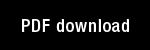Global ratio limit theorems for some nonlinear functional differential equations, I

Author(s): Grossberg, S. |

Year: 1968

Citation: Bulletin of the American Mathematical Society, 74, 93-100

Abstract: 1. Introduction. We study some systems of nonlinear functional-differential equations of the form(1)X(t)= A X(1) + B(Xi)X(t - r) + CO), t' 0,where X=(xi,, xâ€ž) is nonnegative, B(Xj) =jjB;j(t)jj is a matrixof nonlinear functionals of X (w) evaluated at all past times wE [-7-,t],and C= (Cl, â€˘â€˘â€˘ , Câ€ž) is a known nonnegative and continuous inputfunction. For appropriate A, B, and C, these systems can be interpreted as a nonstationary prediction theory whose goal is to discussthe prediction of individual events, in a fixed order, and at prescribed times, or alternatively as a mathematical learning theory.This interpretation is discussed in a special case in [11. The systemscan also be interpreted as cross-correlated flows on networks, or asdeformations of probabilistic graphs.
The mathematical content of these interpretations is contained inassertions of the following kind: given arbitrary positive and continu-ous initial data along with a suitable input C, the ratios yjk(t)=P'j(t) have limits as t-->-. Our systems are defined in the following way. Given any positiveinteger it; any real numbers a, it, 0>0, and r>0; and any nXnsenustochastic matrix [...] In order that our theorems hold, the initial data must always be non

Topics: Mathematical Foundations of Neural Networks, Models: Other,### Cross References

Global ratio limit theorems for some nonlinear functional differential equations, II

Author(s): Grossberg, S. |

Year: 1968

Citation: Bulletin of the American Mathematical Society, 74, 101-105

Abstract: Introduction: A previous note [l] introduced some systems of nonlinear functional-differential equations of the form X(t) = AX(t) + B(Xt)X(t - r) + C(t) i ÂŁ 0, where X~(xi, â€˘ - * , xn) is nonnegative, B(Xt) is a matrix of nonÂ¬linear functionals of X(w) evaluated at all past times Â«/ÂŁ[â€”T, /], and Câ€” (Ci, * â€˘ â€˘ , CÂ«) is a nonnegative and continuous input funcÂ¬tion. Some global ratio limit theorems were then stated for one of these systems. Here twro other cases are considered. In particular, we study the dependence of the stability properties of (1) on the time lag r.

Topics: Mathematical Foundations of Neural Networks, Models: Other,